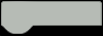# Arduino matrix scrolling weather station and clock with BME 280 and DS3231

From one of my previous projects (Bluetooth-controlled scrolling text), I have a finished 8x56 LEDs matrix consisting of 7pcs MAX7219 Led matrix modules 8x8, and located in the appropriate box. I used this ready-made matrix to make a scrolling weather station that displays the temperature, air humidity and relative atmospheric pressure, as well as the current time.

For this purpose, I use a BME280 sensor and a DS3231 real-time clock module, so the device works independently of an Internet connection. The BME280 board is placed on the outer side of the case for more accurate readings, and it can also be placed outside with the help of a four-wire cable. Let me mention that these matrix modules are older type, and as you see (in the given picture), contain DIL IC on the front. The new modules are made in smd technology and usually composed of 4 coupled matrices, and they are turned 90 degrees clockwise.In this project, using the library "Max72xxPanel" the position of the contents of each matrix can be rotated in code with command "matrix.setRotation", so we can use matrices of any type. Also the number of matrices in the array can be changed, and is defined by the command:
int numberOfHorizontalDisplays = 7;  //we can simple change this numberaccording to number of matrices we use

As I mentioned before, the device is very simple to build and contains the following components:

HARDWARE LIST
1 Arduino nanao
1 DS3231 realtime clock module
1 DFRobot Gravity: I2C BME280 Environmental Sensor
7 Led dot matrix display with MAX7219 controller, RedDue to the large number of diodes, the device should be powered by an external source that should provide a current of 2 Amps or more.
The intensity of the LEDs, as well as the speed of the scrolling text, can be easily adjusted in code.
int wait = 25; // speed
matrix.setIntensity(3); // Use a value between 0 and 15 for brightness
On the video you can watch a short description of how this device is made.And finally, the device is built into a suitable housing made of PVC board with a thickness of 3mm and 5mm

Schematic diagram:Arduino Code

CODE
``````#include <SPI.h>
#include <Max72xxPanel.h>
#include "RTClib.h"

RTC_DS1307 rtc;

char daysOfTheWeek = {"Sunday", "Monday", "Tuesday", "Wednesday", "Thursday", "Friday", "Saturday"};

int mm;

int pinCS = 10; // Uno or Duemilanove DIN 11 (MOSI) CLK 13 (SCK)
int numberOfHorizontalDisplays = 7;
int numberOfVerticalDisplays = 1;

Max72xxPanel matrix = Max72xxPanel(pinCS, numberOfHorizontalDisplays, numberOfVerticalDisplays);

String tape = "";
int wait = 25; // speed

int spacer = 2; // Character spacing (points)
int width = 5 + spacer; // Font width 5 pixels

String utf8rus(String source)
{
int i,k;
String target;
unsigned char n;
char m = { '0', '\0' };

k = source.length(); i = 0;
while (i < k) {
n = source[i]; i++;
if (n >= 0xC0) {
switch (n) {
case 0xD0: {
n = source[i]; i++;
if (n == 0x81) { n = 0xA8; break; }
if (n >= 0x90 && n <= 0xBF) n = n + 0x2F;
break;
}
case 0xD1: {
n = source[i]; i++;
if (n == 0x91) { n = 0xB7; break; }
if (n >= 0x80 && n <= 0x8F) n = n + 0x6F;
break;
}
}
}
m = n; target = target + String(m);
}
return target;
}

unsigned char c; // variable port for reading series
String Serial_string = ""; // Forming from a character string
while (Serial.available() > 0) { // If there are symbols in the series
//Serial.print(c,HEX); Serial.print(" "); Serial.print(c);
if (c == '\n') {  //If this is a string of lines
return Serial_string; // We return the line
}
if (c == 0xB8) c = c - 0x01;
if (c >= 0xBF && c <= 0xFF) c = c - 0x01;
Serial_string = Serial_string + String(char(c)); //Add symbol to line
}
return Serial_string;
}
String chas;
String myn;
//String mesyc = "";

void setup() {

Serial.begin(9600);
Serial.println(F("bme280"));
//==================================== hours
if (! rtc.begin()) {
Serial.println("Couldn't find RTC");
Serial.flush();
abort();
}
if (! rtc.isrunning()) {
Serial.println("RTC is NOT running, let's set the time!");
// When time needs to be set on a new device, or after a power loss, the
// following line sets the RTC to the date & time this sketch was compiled
// This line sets the RTC with an explicit date & time, for example to set
// January 21, 2014 at 3am you would call:
// rtc.adjust(DateTime(2014, 1, 21, 3, 0, 0));
}
//====================================
Serial.println(F("Could not find a valid bme280 sensor, check wiring!"));
delay(2000);
}

matrix.setIntensity(3); // Use a value between 0 and 15 for brightness
matrix.setRotation(matrix.getRotation()+0); //1 - 90  2 - 180   3 - 270
//a=a+1;
}
void loop() {
DateTime now = rtc.now();
pressure = pressure + 78.5  ;   //Relative pressure for 700m above sea level, comment this row for relative atmospheric pressure
//======================================= correction digit time zero before the number
chas ="";
myn = "";
if (now.hour() < 10) {
chas = '0';
}

if (now.minute() < 10) {
myn = ('0');
}

//=======================================
tape = utf8rus((String)+daysOfTheWeek[now.dayOfTheWeek()]+"     Time  "+chas +now.hour()+":"+myn+now.minute()+" h    Temperature = "+temperature +" degree C   Humidity = " + humidity +  " %    Pressure = "+ pressure +" Hpa");
if (Serial.available()){
}
for ( int i = 0 ; i < width * tape.length() + matrix.width() - 1 - spacer; i++ )
{
matrix.fillScreen(LOW);

int letter = i / width; // number of symbols displayed on a matrix

int y = (matrix.width() - 1) - i % width;
int x = (matrix.height() - 8) / 2;

while ( y + width - spacer >= 0 && letter >= 0 ) {
if ( letter < tape.length() ) {
matrix.drawChar(y, x, tape[letter], HIGH, LOW,1);
}
letter--;
y -= width;
}
matrix.write(); // Send a picture for display
delay(wait);
}
}``````